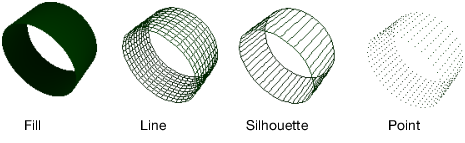Publication date: 08/13/2020

## Primitives Example

The following short example illustrates the use of a graphics primitive.

`shape = Scene Display List(); // create a display list and send it commands`
`shape << Color( 1, 0, 0 ); // set the RGB color of the text`
`shape << Begin( POLYGON );`
`shape << Vertex( 0, 0, 0 );`
`shape << Vertex( 0, 3, 0 );`
`shape << Vertex( 3, 3, 0 );`
`shape << Vertex( 5, 2, 0 );`
`shape << Vertex( 4, 0, 0 );`
`shape << Vertex( 2, -1, 0 );`
`shape << End();`
` `

// draw the window and send it the stored display list

`scene = Scene Box( 400, 400 ); // make a scene box`
`New Window( "Primitive", scene ); // put the scene in a window`
`scene << Perspective( 90, 3, 7 ); // define the camera`
`scene << Translate( 0.0, 0.0, -5 ); // move to (0,0,-5) to draw`
`scene << Call List( shape ); // send the display list to the scene`
`scene << Update; // update the scene`

The first section of the script creates a display list named shape. Inside this display list, a polygon is defined using six vertices.

The second section of the script creates a scene box and a new window. It then uses the Call List message to put the list in the display.

Note that all the z-coordinates are zero, which makes sure the polygon lies in a plane. Polygons that do not lie in a plane can cause unpredictable results.

Experiment with the line

`shape <<Begin(POLYGON);`

by changing it to some of the other primitive types. For example, changing it to

`shape <<Begin(TRIANGLES);`

results in a different picture.

Figure 13.10 Polygon (left) and Triangles (right)